Processing ......FreeComputerBooks.com Free Computer, Mathematics, Technical Books and Lecture Notes, etc.

Theory of Computation and ComputingRelated Book Categories:
•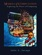Models of Computation: Exploring the Power of Computing

It covers the traditional topics of formal languages, automata and complexity classes, as well as an introduction to the more modern topics of space-time tradeoffs, memory hierarchies, parallel computation, the VLSI model, and circuit complexity.

•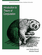Introduction to Theory of Computation (Anil Maheshwari, et al)

This is a textbook on formal languages, automata theory, and computational complexity. This text strikes a good balance between rigor and an intuitive approach to computer theory.

•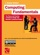Computing Fundamentals: Theory and Practice of Software Design

The book introduces the reader to computer programming, i.e. algorithms and data structures. It covers many new programming concepts that have emerged in recent years including object-oriented programming and design patterns.

•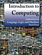Introduction to Computing: Language, Logic, and Machines

This book focuses on how to describe information processes by defining procedures, how to analyze the costs required to carry out a procedure, and the fundamental limits of what can and cannot be computed mechanically.

•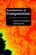Foundations of Computation (Carol Critchlow, et al)

It includes topics from discrete mathematics, automata theory, formal language theory, and the theory of computation, along with practical applications to computer science. The course has no prerequisites other than introductory computer programming.

•Introduction to Theory of Computation (Eitan M. Gurari)

This book explores some of the more important terminologies and questions concerning programs, computers, problems, and computation. The exploration reduces in many cases to a study of mathematical theories, such as those of automata and formal languages; theories that are interesting also in their own right.

•Applicative Computing: Its Quarks, Atoms and Molecules

This work covers the advanced topics in main ideas of computing in general. Material is especially useful for the instructor, postgraduate and graduate students of IT-specialties and is suitable for the system of training of specialists.

•Matters Computational: Ideas, Algorithms, Source Code

This book provides algorithms and ideas for computationalists, whether a working programmer or anyone interested in methods of computation. The implementations are done in C++ and the GP language, written for POSIX-compliant platforms.

•Introduction to Soft Computing (Eva Volna)

This book gives an introduction to Soft Computing, which aims to exploit tolerance for imprecision, uncertainty, approximate reasoning, and partial truth in order to achieve close resemblance with human like decision making.

•Applicative Computing (Jimmy Or)

This book provides an overview of state of the art research in Affective Computing. It presents new ideas, original results and practical experiences in this increasingly important research field.

•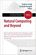Natural Computing and Beyond (Yasuhiro Suzuki, et al)

This book compiles refereed contributions to various aspects of Natural Computing, ranging from computing with slime mold, artificial chemistry, eco-physics, and synthetic biology, to computational aesthetics.

•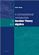A Computational Introduction to Number Theory and Algebra

This introductory book emphasises algorithms and applications, such as cryptography and error correcting codes, and is accessible to a broad audience.

•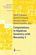Computations in Algebraic Geometry with Macaulay 2 (D.Eisenbud)

This book presents algorithmic tools for algebraic geometry and experimental applications of them using Macaulay 2.

•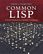Common LISP: A Gentle Introduction to Symbolic Computation

This highly accessible introduction to Lisp is suitable both for novices approaching their first programming language and experienced programmers interested in exploring a key tool for artificial intelligence research.

•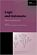Logic and Automata: History and Perspectives (Jorg Flum, et al)

This book gives a consolidated overview of the research results achieved in the theory of automata and logic, covers many different facets of logic and automata theory, emphasizing the connections to other disciplines such as games, algorithms, etc.

•Logics of Time and Computation (Robert Goldblatt)

This is a short but excellent introduction to modal, temporal, and dynamic logic, etc. It manages to cover, in highly readable style, the basic completeness, decidability, and expressability results in a variety of logics of the three kinds considered.

•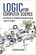Logic for Computer Science: Automatic Theorem Proving

This book introduces mathematical logic with an emphasis on proof theory and procedures for algorithmic construction of formal proofs. It is useful for the formalization of proofs and basics of automatic theorem proving.

•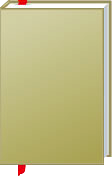Theory of Computation and Computing

This is the previous page of Theory of Computation and Computing, we are in the processing to convert all the books there to the new page. Please check this page again!!!

Book CategoriesAll CategoriesRecent BooksIT Research LibraryMiscellaneous Books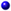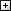Computer LanguagesComputer ScienceData Science/DatabasesElectronic EngineeringJava and Java EE (J2EE)Linux and UnixMathematicsMicrosoft and .NETMobile ComputingNetworking and CommunicationsSoftware EngineeringSpecial TopicsWeb Programming
Other Categories